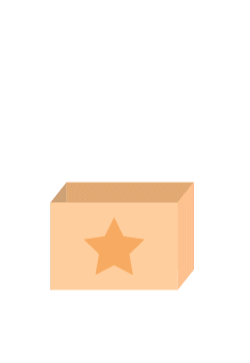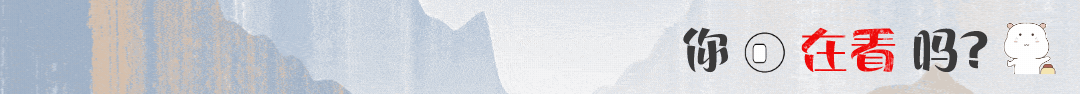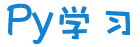注册    登录创作新主题

Python
 python开源   Django   Python   DjangoApp   pycharm
DATA
 docker   Elasticsearch

 问与答   闲聊   招聘   翻译   创业   分享发现   分享创造   求职   区块链   支付之战
aigc
 aigc   chatgpt
WEB开发
 linux   MongoDB   Redis   DATABASE   NGINX   其他Web框架   web工具   zookeeper   tornado   NoSql   Bootstrap   js   peewee   Git   bottle   IE   MQ   Jquery

Python88.com
 反馈   公告   社区推广

Py学习  »  Python

# 8个流行的Python可视化工具包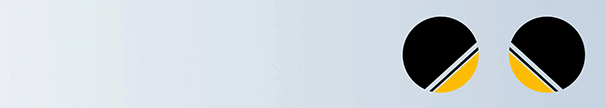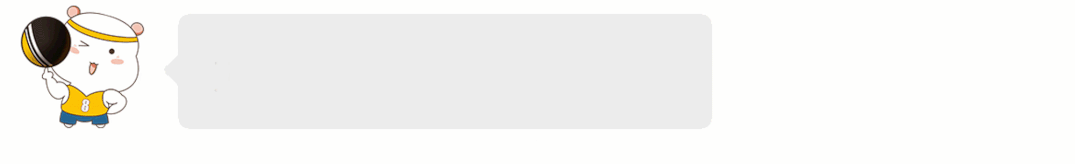## Matplotlib、Seaborn 和 Pandas

Matplotlib 是比较低级的库，但它所支持的自定义程度令人难以置信（所以不要简单地将其排除在演示所用的包之外！），但还有其它更适合做展示的工具。

Matplotlib 还可以选择样式（style selection），它模拟了像 ggplot2 和 xkcd 等很流行的美化工具。下面是我用 Matplotlib 及相关工具所做的示例图：

``import seaborn as snsimport matplotlib.pyplot as pltcolor_order = ['xkcd:cerulean', 'xkcd:ocean',                'xkcd:black','xkcd:royal purple',                'xkcd:royal purple', 'xkcd:navy blue',                'xkcd:powder blue', 'xkcd:light maroon',                 'xkcd:lightish blue','xkcd:navy']sns.barplot(x=top10.Team,            y=top10.Salary,            palette=color_order).set_title('Teams with Highest Median Salary')plt.ticklabel_format(style='sci', axis='y', scilimits=(0,0))``

``import matplotlib.pyplot as pltimport scipy.stats as stats#model2 is a regression modellog_resid = model2.predict(X_test)-y_teststats.probplot(log_resid, dist="norm", plot=plt)plt.title("Normal Q-Q plot")plt.show()``

Seaborn 绘制 21 种超实用精美图表

## ggplot(2)

ggplot2（我觉得也包括 Python 的 ggplot）举足轻重的原因是它们用「图形语法」来构建图片。基本前提是你可以实例化图，然后分别添加不同的特征；也就是说，你可以分别对标题、坐标轴、数据点以及趋势线等进行美化。

``#All Salariesggplot(data=df, aes(x=season_start, y=salary, colour=team)) +  geom_point() +  theme(legend.position="none") +  labs(title = 'Salary Over Time', x='Year', y='Salary (\$)')``

## Bokeh

Bokeh 很美。从概念上讲，Bokeh 类似于 ggplot，它们都是用图形语法来构建图片，但 Bokeh 具备可以做出专业图形和商业报表且便于使用的界面。为了说明这一点，我根据 538 Masculinity Survey 数据集写了制作直方图的代码：

``import pandas as pdfrom bokeh.plotting import figurefrom bokeh.io import show# is_masc is a one-hot encoded dataframe of responses to the question:# "Do you identify as masculine?"#Dataframe Prepcounts = is_masc.sum()resps = is_masc.columns#Bokehp2 = figure(title='Do You View Yourself As Masculine?',          x_axis_label='Response',          y_axis_label='Count',          x_range=list(resps))p2.vbar(x=resps, top=counts, width=0.6, fill_color='red', line_color='black')show(p2)#Pandascounts.plot(kind='bar')``

Bokeh 提供的所有便利都要在 matplotlib 中自定义，包括 x 轴标签的角度、背景线、y 轴刻度以及字体（大小、斜体、粗体）等。下图展示了一些随机趋势，其自定义程度更高：使用了图例和不同的颜色和线条。

Bokeh 还是制作交互式商业报表的绝佳工具。详情点点击查看👇
Python Bokeh 库进行数据可视化实用指南

## Plotly

Plotly 非常强大，但用它设置和创建图形都要花费大量时间，而且都不直观。在用 Plotly 忙活了大半个上午后，我几乎什么都没做出来，干脆直接去吃饭了。我只创建了不带坐标标签的条形图，以及无法删掉线条的「散点图」。Ploty 入门时有一些要注意的点：

• 安装时要有 API 秘钥，还要注册，不是只用 pip 安装就可以；
• Plotly 所绘制的数据和布局对象是独一无二的，但并不直观；
• 图片布局对我来说没有用（40 行代码毫无意义！）

• 你可以在 Plotly 网站和 Python 环境中编辑图片；
• 支持交互式图片和商业报表；
• Plotly 与 Mapbox 合作，可以自定义地图；
• 很有潜力绘制优秀图形。

``#plot 1 - barplot# **note** - the layout lines do nothing and trip no errorsdata = [go.Bar(x=team_ave_df.team,              y=team_ave_df.turnovers_per_mp)]layout = go.Layout(    title=go.layout.Title(        text='Turnovers per Minute by Team',        xref='paper',        x=0    ),    xaxis=go.layout.XAxis(        title = go.layout.xaxis.Title(            text='Team',            font=dict(                    family='Courier New, monospace',                    size=18,                    color='#7f7f7f'                )        )    ),    yaxis=go.layout.YAxis(        title = go.layout.yaxis.Title(            text='Average Turnovers/Minute',            font=dict(                    family='Courier New, monospace',                    size=18,                    color='#7f7f7f'                )        )    ),    autosize=True,    hovermode='closest')py.iplot(figure_or_data=data, layout=layout, filename='jupyter-plot', sharing='public', fileopt='overwrite')#plot 2 - attempt at a scatterplotdata = [go.Scatter(x=player_year.minutes_played,                  y=player_year.salary,                  marker=go.scatter.Marker(color='red',                                          size=3))]layout = go.Layout(title="test",                xaxis=dict(title='why'),                yaxis=dict(title='plotly'))py.iplot(figure_or_data=data, layout=layout, filename='jupyter-plot2', sharing='public')[Image: image.png]``

## Pygal

Pygal 的名气就不那么大了，和其它常用的绘图包一样，它也是用图形框架语法来构建图像的。由于绘图目标比较简单，因此这是一个相对简单的绘图包。使用 Pygal 非常简单：

• 实例化图片；
• 用图片目标属性格式化；

## Networkx

``````options = {    'node_color' : range(len(G)),    'node_size' : 300,    'width'

: 1,    'with_labels' : False,    'cmap' : plt.cm.coolwarm}nx.draw(G, **options)``````

``import itertoolsimport networkx as nximport matplotlib.pyplot as pltf = open('data/facebook/1684.circles', 'r')circles = [line.split() for line in f]f.close()network = []for circ in circles:    cleaned = [int(val) for val in circ[1:]]    network.append(cleaned)G = nx.Graph()for v in network:    G.add_nodes_from(v)edges = [itertools.combinations(net,2) for net in network]for edge_group in edges:    G.add_edges_from(edge_group)options = {    'node_color' : 'lime',    'node_size' : 3,    'width' : 1,    'with_labels' : False,}nx.draw(G, **options)``

https://towardsdatascience.com/reviewing-python-visualization-packages-fa7fe12e622b

4W字，最强 Matplotlib 实操指南！
Seaborn 绘制 21 种超实用精美图表

Python Bokeh 库进行数据可视化实用指南

Python 绘制惊艳的瀑布图

Python地图绘制工具folium基础知识全攻略